Thursday, October 28, 2021
HomeData ScienceData Science vs. Machine Learning: 15 Best Things You Need To Know

# Data Science vs. Machine Learning: 15 Best Things You Need To Know

We observe the contribution of artificial intelligence, data science, and machine learning in modern technology like the self-driving car, ride sharing app, smart personal assistant, and so forth. So, these terms are now buzzwords for us that we talk about these all the time, but we don’t understand these in depth. Also, as a layman, these are complex terms for us. Though data science covers machine learning, there is a distinction between data science vs. machine learning from insight. In this article, we have described both of these terms in simple words. So, you can get a clear idea of these fields and distinctions between them. Before going into the details, you might be interested in my previous article, which is also closely related to data science – Data Mining vs. Machine Learning.

## Data Science vs. Machine Learning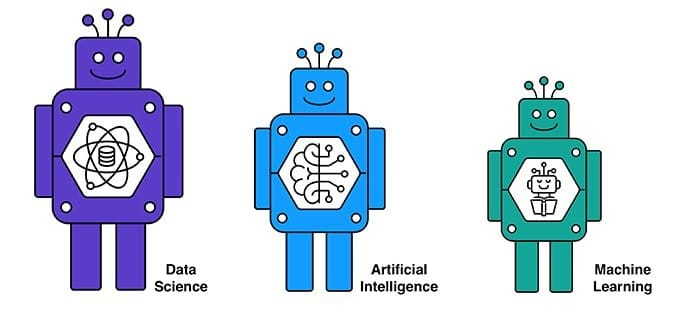Data science is a process of extracting information from unstructured/raw data. To accomplish this task, it uses several algorithms, ML techniques, and scientific approaches. Data science integrates Statistics, Machine Learning, and Data Analytics. Below we are narrating 15 distinctions between Data Science vs. Machine Learning. So, let’s start.

### 1. Definition of Data Science & Machine Learning

Data Science is a multi-disciplinary approach which integrates several fields and applies scientific methods, algorithms, and processes to extract knowledge and draw meaningful insights from structured and unstructured data. This board field covers a wide range of domains, including Artificial Intelligence, Deep Learning, and Machine Learning. The objective of data science is to describe the meaningful insights of data.

Machine Learning is the study of developing an intelligent system. Machine learning makes a machine or device able to learn, identify patterns, and make a decision automatically. It uses algorithms and mathematical models to make the machine intelligent and autonomous. It makes a machine able to perform any task without explicitly programmed.

In a word, the main difference between data science vs. machine learning is that data science covers the entire data processing process, not just the algorithms. The main concern of machine learning is algorithms.

### 2. Input Data

The input data of data science is human readable. The input data can be tabular form or images which can be read or interpreted by a human. The input data of machine learning is processed data as the requirement of the system. The raw data is pre-processed using specific techniques. As an instance, feature scaling.

### 3. Data Science & Machine Learning Components

The components of data science include the collection of data, distributed computing, automatic intelligence, visualization of data, dashboards, and BI, data engineering, deployment in production mood, and an automated decision.

On the other hand, machine learning is the process of developing an automatic machine. It starts with data. The typical components of machine learning components are problem understanding, explore data, prepare data, model selection, train the system.

### 4. Scope of Data Science & ML

Data science can be applied to almost all real-life problems wherever we need to draw insights from data. The tasks of data science include understanding the system requirements, extraction of data, and so forth.

Machine learning, on the other hand, can be applied where we need to classify accurately or predict the outcome for new data by learning the system using a mathematical model. Since the present era is the era of artificial intelligence, so machine learning is very demanding for its autonomous capability.

### 5. Hardware Specification for Data Science & ML Project

Another primary distinction between data science and machine learning is the specification of hardware. Data science requires horizontally scalable systems to handle the vast amount of data. High-quality RAM and SSD is needed to avoid the problem of I/O bottleneck. On the other hand, in machine learning GPUs are required for intensive vector operations.

### 6. System Complexity

Data science is an interdisciplinary field that is used to analyze and extract vast amounts of unstructured data and provide significant insight. The system’s complexity depends on the massive amount of unstructured data. On the contrary, the complexity of the machine learning system depends on the algorithms and mathematical operations of the model.

### 7. Performance Measure

The performance measure is such an indicator which indicates how much a system can perform its task accurately. It is one of the crucial factors to differentiate data science vs. machine learning. In terms of data science, the factor performance measure is not standard. It varies problem by problem. Generally, it is an indication of data quality, querying ability, the effectiveness of data access, and user-friendly visualization, etc.

As opposed to, in terms of machine learning, the performance measure is standard. Every algorithm has a measure indicator which can describe is the model fits for the given training data and the error rate. As an instance, Root Mean Square Error is used in Linear Regression to determine the error in the model.

### 8. Development Methodology

The development methodology is one of the critical distinctions between data science vs. machine learning. The development methodology of a data science project is like an engineering task. On the contrary, the machine learning project is a research-based task, where with the help of data, a problem is solved. A machine learning expert has to evaluate its model again and again to enhance its accuracy.

### 9. Visualization

Visualization is another significant difference between data science and machine learning. In data science, visualization of data is done using graphs such as pie chart, bar chart, etc. However, in machine learning visualization is used to express a mathematical model of training data. As an instance, in a multi-class classification problem, the visualization of a confusion matrix is used to determine false positives and negatives.

### 10. Programming Language for Data Science & ML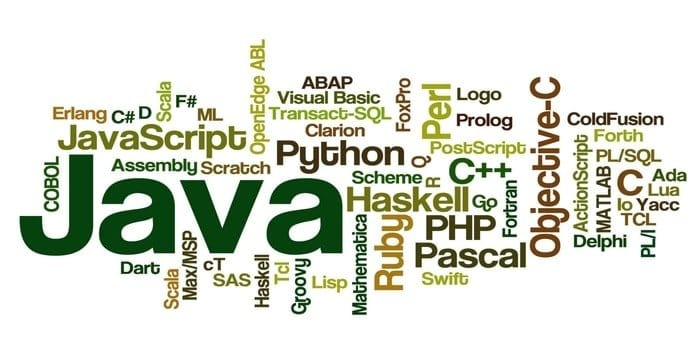Another key difference between data science vs. machine learning is how they are programmed or what kind of programming language they are used. To solve the data science problem, SQL and SQL like syntax, i.e., HiveQL, Spark SQL is the most popular.

Perl, sed, awk can also be used as data processing scripting language. Furthermore, a framework supported languages (Java for Hadoop, Scala for Spark) are widely used for coding data science problem.

Machine learning is the study of algorithms that enables a machine to learn and take action by its. There are several machine learning programming language. Python and R are the most popular programming language for machine learning. There is more in addition to these such as Scala, Java, MATLAB, C, C++, and so forth.

### 11. Preferred Skillset: Data Science & Machine Learning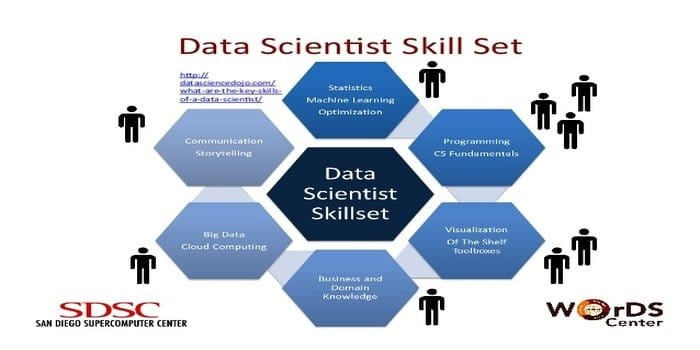A data scientist is responsible for collecting and manipulating the massive amount of raw data. The preferred skillset for data science is:

• Data Profiling
• ETL
• Expertise in SQL
• Ability to handle unstructured data

On the contrary, the preferred skillset for Machine Learning is:

• Critical Thinking
• Strong mathematical and statistical operations understanding
• Good knowledge in the programming language, i.e., Python, R
• Data processing with SQL model

### 12. Data Scientist’s Skill vs. Machine Learning Expert’s Skill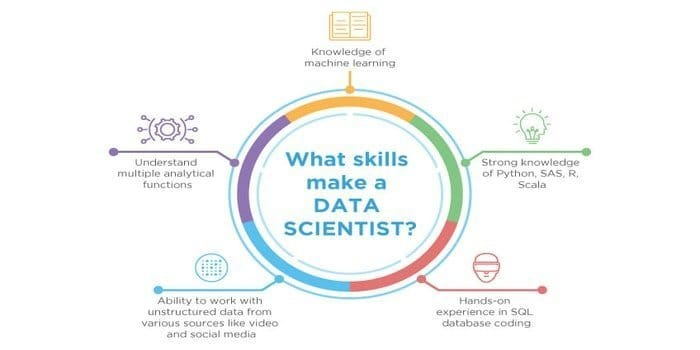As, both the data science and machine learning are the potential fields. Therefore, the job sector is proliferating. The skills of both fields may intersect, but there is a difference between both of them. A data scientist must need to know:

• Data mining
• Statistics
• SQL databases
• Unstructured data management techniques
• Big data tools, i.e., Hadoop
• Data visualization

On the other side, a machine learning expert must need to know:

### 13. Workflow: Data Science vs. Machine Learning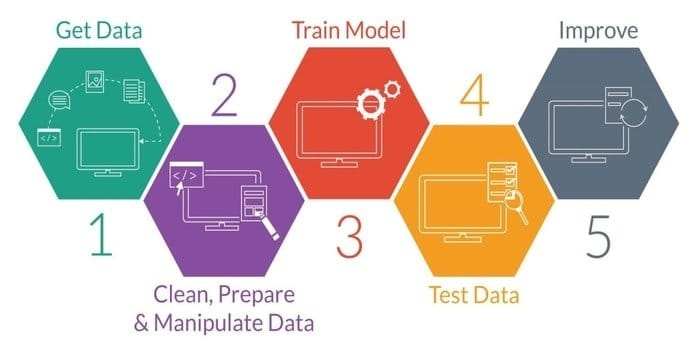Machine learning is the study of developing an intelligent machine. It provides the machine such a capability that it can act without explicitly programmed. To develop an intelligent machine, it has five stages. They are as follows:

• Import Data
• Data Cleansing
• Model Building
• Training
• Testing
• Improve the model

The concept of data science is used to handle big data. The responsibility of a data scientist is to collect data from multiple sources and apply several techniques to extract information from the dataset. The workflow of data science has the following stages:

• Requirements
• Data Acquisition
• Data Processing
• Data Exploration
• Modeling
• Deployment

Machine learning helps data science by providing algorithms for data exploration and, and so forth. On the contrary, data science combines machine learning algorithms to predict the outcome.

### 14. Application of Data Science & Machine Learning

Nowadays, data science is one of the most popular fields worldwide. Its a necessity for industries and therefore, several applications are available in data science. Banking is one of the most significant areas of data science. In banking, data science is used for fraud detection, customer segmentation, predictive analysis, etc.

Data science is also used in finance to customer data management, risk analytics, consumer analytics, etc. In healthcare, data science is used to medical analysis image, drug discovery, monitoring patient health, preventing diseases, tracking diseases, and many more.

On the other side, machine learning is applied in various domains. One of the most splendid applications of machine learning is image recognition. Another use is speech recognition that is the translation of spoken words into text. There are more applications in addition to these like video surveillance, self-driving car, text to emotion analyzer, author identification, and many more.

Machine learning is also used in healthcare for heart disease diagnosis, drug discovery, robotic surgery, personalized treatment, and many more. Additionally, machine learning is also used for information retrieval, classification, regression, prediction, recommendations, natural language processing, and many more.

### 15. Tools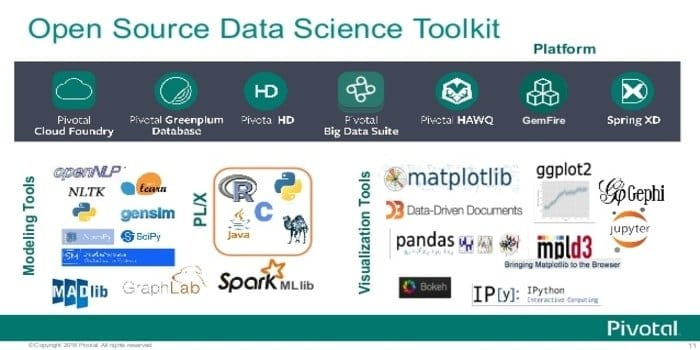The responsibility of a data scientist is to extract information, manipulate and pre-process data. On the other hand, in a machine learning project, the developer needs to build an intelligent system. So, the function of both disciplines is different. Therefore, the tools they are used to develop their project is different from each other though there are some common tools.

Several tools are used in data science. SAS, a data science tool, is used to perform statistical operations. Another popular data science tool is BigML. In data science, MATLAB is used to simulate neural networks and fuzzy logic. Excel is another most popular data analysis tool. There is more in addition to these like ggplot2, Tableau, Weka, NLTK, and so forth.

There are several machine learning tools are available. The most popular tools are Scikit-learn: written in Python and easy to implement machine learning library, Pytorch: an open deep-learning framework, Keras, Apache Spark: an open-source platform, Numpy, Mlr, Shogun: an open source machine learning library.

## Ending Thoughts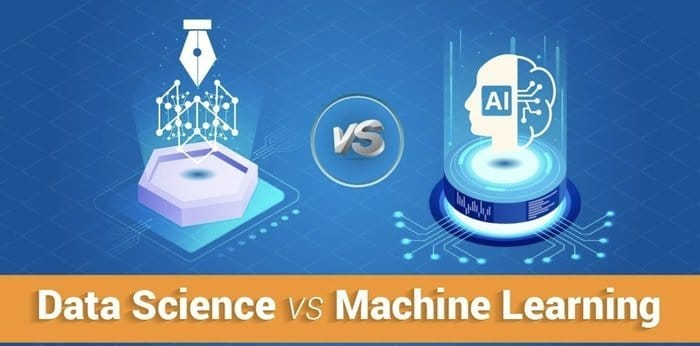Data science is an integration of multiple disciplines, including machine learning, software engineering, data engineering, and many more. Both of these two fields try to extract information. However, machine learning uses various techniques like supervised machine learning approach, unsupervised machine learning approach. On the contrary, data science does not use this type of process. Hence, the main difference between data science vs. machine learning is that data science not only concentrates on algorithms but also the whole data processing. In one word, data science and machine learning both are the two demanding fields that are used to solve a real-world problem in this technology-driven world.